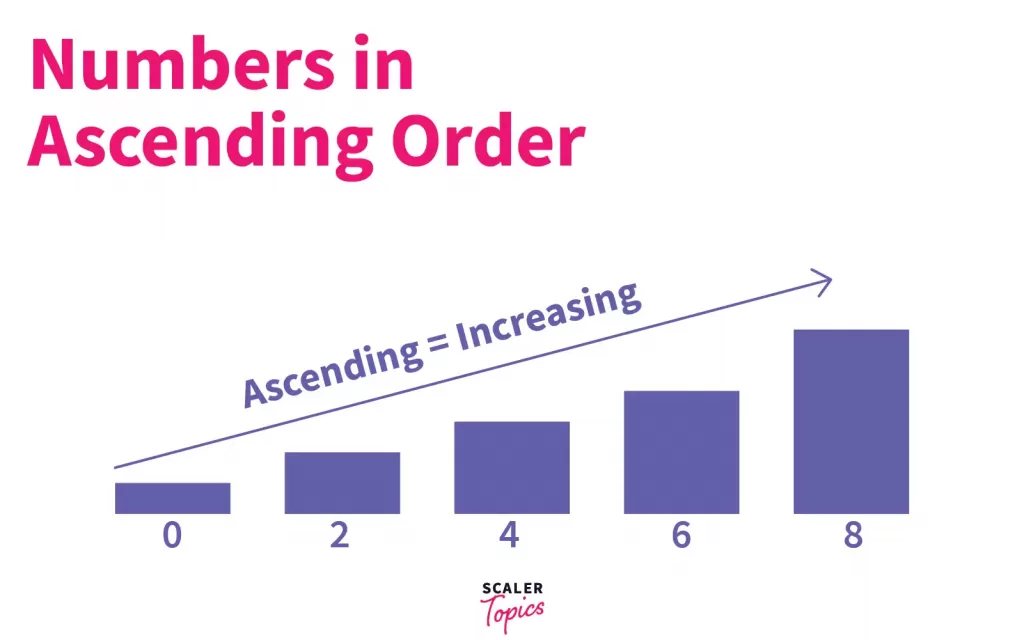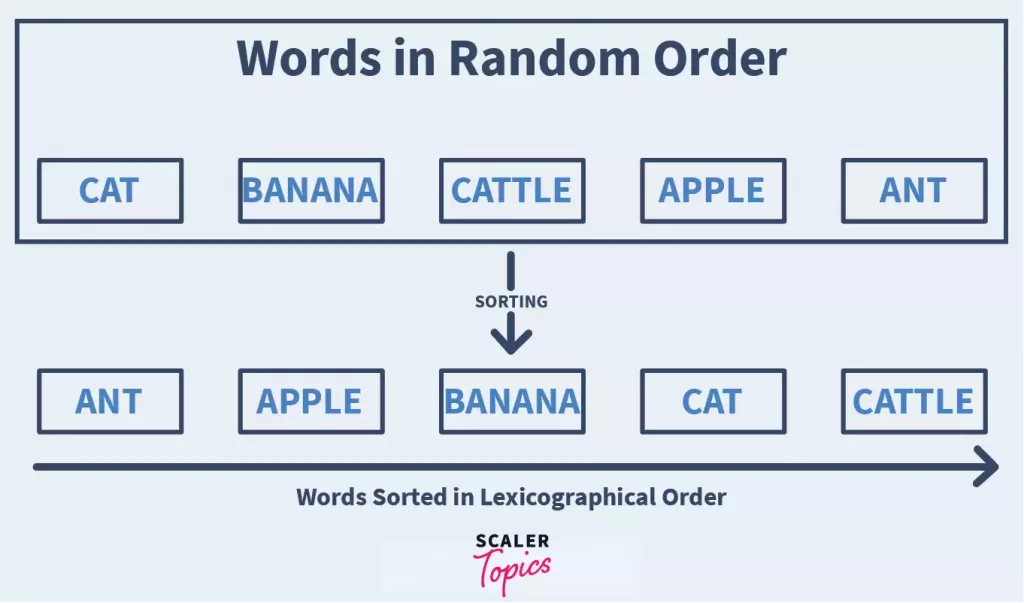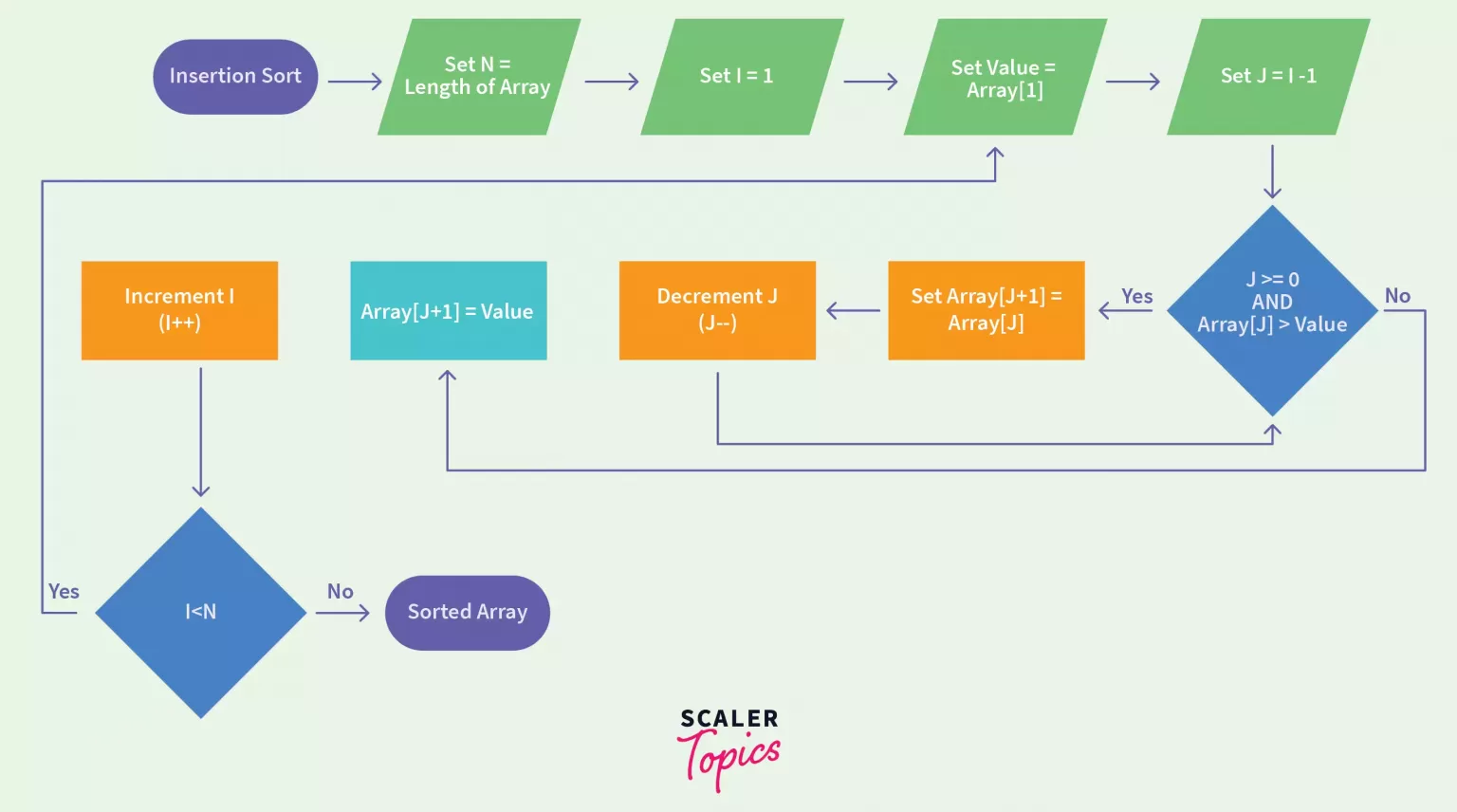# Insertion Sort

Challenge Inside! : Find out where you stand! Try quiz, solve problems & win rewards!
Video Tutorial## Overview

Insertion sort is a sorting algorithm in which the elements are transferred one at a time to the right position.

## Introduction

"Sorting is a way of arranging the elements in a certain order".

The most frequently used orders are numerical order(where numbers are sorted in increasing or decreasing order) or lexicographical order(like words are sorted in a dictionary).

### Numbers Sorted in Increasing Order### Sorting Example for WordsAs you might have guessed, sorting is very useful for optimizing the efficiency of other algorithms. For instance, sorting plays a very critical role in searching algorithms.

Consider a case of a school where we want to assign roll numbers to the students. We can sort them by their names and assign roll numbers in an increasing manner. It would be a lexicographical order sorting, where we are sorting by words.

At the same time, to find the top N students with the highest marks in some subject, we can basically sort the students by their marks to find top N among them. This would be numerical order sorting.

So we can see how sorting plays an important role in our daily lives.

## What is Insertion Sort?

Insertion Sort is one of the simplest sorting techniques which you might have used in your daily lives while arranging a deck of cards.

So without going into how this algorithm works, let’s think about how you would usually go about arranging the deck of cards?

Say you are given 10 cards, 1 to 10 of spades, all shuffled, and you want to sort these cards.

• You would basically pick any random card(e.g. 7), and place it into your left hand, assuming the left hand is meant to carry the sorted cards.
• Then you would pick another random card, say 2, and place 2 in the correct position on your left hand, i.e. before 7.
• Then again if you pick 5, you would place it between 2 and 7 on your left hand, and this way we know we are able to sort our deck of cards. Since we insert one element at a time in its correct position, hence its name “Insertion Sort”.

## Insertion Sort Algorithm

Insertion Sort Pseudocode:

Explanation:

Line 2: We don’t process the first element, as it has nothing to compare against.
Line 3: Loop from i=1 till the end, to process each element.
Line 4: Extract the element at position i i.e. array[i]. Let it be called E.
Line 5: To compare E with its left elements, loop j from i-1 to 0
Line 6, 7: Compare E with the left element, if E is lesser, then move array[j] to right by 1.
Line 8: Once we have found the position for E, place it there.

## Working of Insertion Sort Algorithm

Elaborating on the same idea, let’s try forming a method to feed it to our computers. We pick one element at a time, compare it against all elements on one side of it(e.g. left) and place this into the correct place.

E.g. if elements were in order:

You can start by picking 3, and since there is no element to the left of 3, we can assume it is in the correct place.
Array:

You can pick 5, you compare 5 with 3, and you find 5 is in the correct order amongst the array of [3, 5].
Array:

Then you pick 2, you find the place in the left side array of [3,5] to place this 2. Since 2 must come before 3, we insert 2 before 3.
Array:

Which is a sorted order.

So, if you must notice, whenever we pick a new element, all elements to the left of it are already sorted(highlighted as green). Think of it as analogous to the left hand while sorting the deck of cards, which carries sorted cards. We just compare in the left sorted half to find the place to place this element. We place this at that position and shift all other elements.

If we continue this till the end, we will get up to the last element placed in the correct position, i.e. the entire array will be sorted.

## Insertion Sort Example

Let’s try to understand this with an example, to understand in detail.

Array:

### Processing 9:

Highlighted green elements to the left are always sorted. We begin with the element in position 0 in the sorted portion, and we will be moving the element in position 1 to the left until it is sorted.

Array:

### Processing 6:

Move the element to the left until it reaches the correct position.

Array:

### Processing 7:

Move the element to the left until it reaches the correct position.

Array:

### Processing 2:

Move the element(blue) to the left until it reaches the correct position.

Array:

Since 2 is not in the correct position in the array of [6, 7, 2, 9], keep processing it.

Array:

Since 2 is still in the correct position in the array of [6, 2, 7, 9], keep processing it.

Array:

### Processing 5:

Move the element(blue) to the left until it reaches the correct position.

Array:

Since 5 is not in the correct position in the array of [2, 6, 7, 5, 9], keep processing it.

Array:

Since 5 is still not in the correct position in the array of [2, 6, 5, 7, 9], keep processing it.

Array:

### Processing 8:

Move the element to the left until it reaches the correct position.

Array:

Since 8 was the last element, and we know that elements towards the left of the currently processed element is sorted, we have the entire array sorted when we process the last element.

So we used the Insertion Sort algorithm to sort the given array of [9, 6, 7, 2, 5, 8] to get [2, 5, 6, 7, 8, 9].

## Flowchart of Insertion Sort Algorithm

As explained above, the algorithm picks one element at a time, Say, currentElement = array[i]

Now, since we want to place it into the correct position in the array of elements towards its left, we take j = i-1 (i.e. just one left of the current element at position i).

We compare the current we took above with all Array[j] for j from i-1 to 0. Until currentElement < Array[j], shift the element at j position to j+1 position.

Note that once we place an element to its correct position, we shift all elements greater than this to one step right.

Once the currentElement < Array[j] is not true, means we need not go any more left, we know j is the position where this current element is to be placed. So we do array[j] = currentElement.

Now, if we have done this without shifting the elements, then the original element placed at j would be lost. So we first shift all j to the j+1 position and then place the current element at j.

We will continue to do this for all “i” from 0 to the length of an array.Output:

Output:

Output:

Output:

Output:

Output:

## Insertion Sort Time Complexity

Insertion sort performs two operations: It scans through the list, comparing each pair of elements, and it shifts the elements if they are out of order.

Each operation contributes to the running time of the algorithm.

• Best Case: O(N). The best case for Insertion Sort would occur when the array is already sorted.

In that case, we run a loop i from 1 → N i.e. total N iterations.

But the inner loop which shifts the elements is never run. This is because if the array is sorted in ascending order, there will not be any element arr[j] to the left of currentElement which is more than currentElement. Hence arr[j] > currentElement will never be true, and the inner loop will never execute.

So there will only be N iterations and the time complexity will be Linear i.e. O(N).

• Worst Case: O($N^2$) The worst case is when the array is sorted but in reverse order.

E.g. If we want to arrange the array in ascending order, but the given input has them in descending order, then in this scenario the worst-case complexity occurs in Insertion Sort.

Outer i loop will run for 1 → N.

And for every j from i-1 → 0, we need to do all these comparisons as every element is in reverse.

So that means for every Nth element, (N-1) comparisons are made.

So total number of comparisons = $N * (N-1) = O(N^2)$

#### To Prove Mathematically

In the worst-case scenario, to insert the last element, we need at-most (n-1) comparisons, and at-most (n-1) shifts.

Similarly, to insert the second-last element, we need at most (n-2) comparisons and at-most (n-2) shifts, and so on.

So the number of operations needed to perform Insertion sort is therefore

• Average Case: O($N^2$) The average case is when all the elements in the given input array are in jumbled and mixed-up order, i.e. neither ascending nor descending.

Similar calculations as done for the Worst-case are also applicable for the Average case scenario, resulting in $O(N^2)$ time complexity.

## Insertion Sort Space Complexity

It iterates over every element, extracts that out to a variable, and compares it against all of its left elements.

So only space taken is for that variable.

Since the space utilized by the algorithm does not depend on how big the array is, no matter how big or small the given input array, it will take constant space.

Space Complexity of Insertion Sort Algorithm = O(1).

### Can We Optimize It Further?

Searching for the correct position of an element and Shifting the rest of the elements are two main operations included in the Algorithm.

• We can optimize the search by using Binary Search:

### Brief introduction on Binary Search:

If we have sorted set of elements and search an integer in these, we can either transverse through all these elements one by one until we find the one we are looking for(this method is also called “linear search”), or can use the Binary Search technique which is much faster as we need not transverse through all elements.

In Binary Search, we basically pick the middle element, compare it against the one we are searching, and based on it we can know if the element would lie towards the left or towards the right of this middle element. But please note that this only works if the array is sorted.

E.g. Given sorted set of elements: 4, 6, 8, 9, 11, 12, 15

And we need to find 12.
We first compare 12 against the middle element i.e. 9.
Since 12 > 9, we can find that 12 must lie somewhere towards the right of this 9, as this given set is sorted.
So we follow same idea for next set of elements i.e. right of 9 i.e. 11, 12, 15
Again we compare 12 against the middle of 11, 12, 15 i.e. 12
Since 12 == 12, we have found the element.

If you compare it against the normal search of traversing the entire set of elements one by one, here we emit one-half of all the elements with each comparison, so the number of comparisons required here is much lesser(actually reducing by 2 every time, so $Log_2{N}$) i.e. O(log N) instead of O(N).

So using Binary search to search for elements i-1 to 0, as j is doing:

This will improve the searching complexity from O(n) to O(log n), i.e. Instead of linearly going back from i-1 → 0, we can perform the Binary search to find the position, and this currentElement should be placed.

And for n elements, the searching complexity will be improved to O(N Log N).

But another half of the algorithm i.e. Shifting will still need O(N) time for one element.

And for n elements, it will still go to $O(N^2)$ time.

• We can optimize the shifting by using a Doubly Linked list:

### Brief Introduction on Doubly Linked List:

Versus the arrays where if we insert an element in between given elements, we would basically need to shift all elements by 1 towards right, as it’s happening in insertion sort, which lets it rise to O(N).

These insertions can be significantly improved using Linked List, where every node has a pointer to the next Node, and this way all the elements are linked.

Array:

So to insert say 4 between 1 and 2, we can just adjust pointers of 1 and 2 only, and no other shifting will be required.

So 1’s pointer is changed to a new Node i.e. 4 and 4’s pointer is changed to the previous pointer of 1 i.e. 2.

So as we can see, insertions can be done in constant time in LinkedLists by just changing the pointers of 2 nodes we are inserting this element between.

Now Doubly LinkedList is nothing but a linkedList with 2 pointers, to go towards left and right, so e.g. here from 2 we can go back to 1 and can go forward to 3.

### Using a Doubly Linked list

A Doubly Linked List will improve the complexity of “shifting” from O(N) to O(1) as seen above.

But the complexity to search still remains $O(N^2)$ as we cannot use binary search in linked lists.

That’s because finding the middle element in LinkedList can not be done in O(1) as it can be done in Arrays where we can pick an element at any index.

Since in LinkedList to find the middle element, we need to traverse the Linked List halfway through, so Search operation is still expensive.

Hence, The overall complexity remains $O(N^2)$.

Therefore, we can conclude that we cannot reduce the worst-case time complexity of insertion sort from $O(N^2)$.

## Applications of Insertion Sort

• Since the time complexity of Insertion sort can go to $O(N^2)$, it is only useful when we have a lesser number of elements to sort in an array.
• Insertion sort is an in-place algorithm, meaning it requires no extra space.
• Maintains relative order of the input data in case of two equal values (stable).

## Conclusion

Insertion Sort works best with a small number of elements. The worst-case runtime complexity of Insertion Sort is $O(n^2)$ similar to that of Bubble Sort. However, Insertion Sort is considered better than Bubble sort.

## Takeaways

An insertion sort compares values in turn, starting with the second value in the list. If this value is greater than the value to the left of it, no changes are made. Otherwise, this value is repeatedly moved left until it meets a value that is less than it.

Complexity of Insertion sort

• Time complexity - O($N^2$)
• Space complexity - O(N)